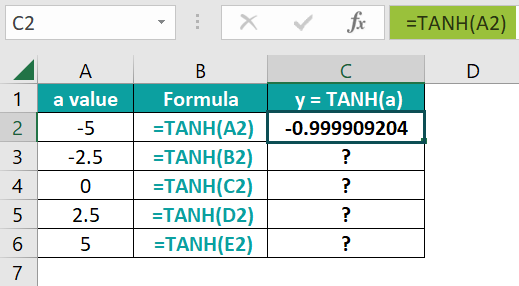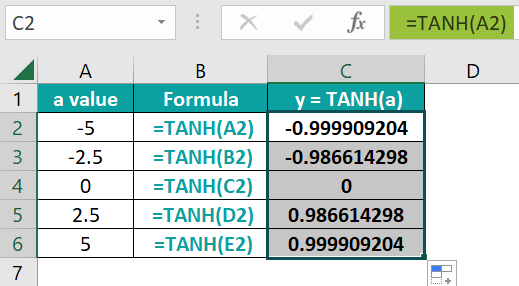# TANH Function in ExcelArticle byExcelMojo Team## What is TANH Function in Excel?

The TANH function calculates the hyperbolic tangent of a number. The function takes a single argument: any positive or negative integer or a decimal number. It belongs to the class of Math and Trig functions in Excel.

Hyperbolic functions such as the TANH function in Excel are used to calculate angles and distances in hyperbolic geometry. Let us look at an example where we must find the TANH value for some numbers. Apply the formula =TANH(A2) in cell B2. Press Enter and drag the Excel Fill handle till B4 to get the TANH values of the three numbers 0, -1, and 0.5. Thus, the function returns the hyperbolic tangent of the numbers, both positive and negative. It lies between the values of -1 and 1.

###### Key Takeaways
• The TANH function in Excel is one of the hyperbolic functions. We get the hyperbolic tangent of any number. It can also be found using the formula TANH(x) = SINH(x)/COSH(x).
• It takes a single argument. The argument can be any integer or decimal number, positive or negative. If a non-numeric argument is supplied, we get an #VALUE error. If the argument is in degrees, you should convert it to radians to get the hyperbolic tangent.
• The function simplifies complex calculations involving neural networks, electromagnetic theory, heat transfer, etc.

### TANH() Excel Formula

The TANH function in Excel has the following syntax:

Arguments: The function has only one argument, which is mandatory.

• Number (mandatory): A real number

Mathematically, the TANH formula is as follows:

### How to Use TANH Function in Excel?

We can use the TANH function in two ways. It can be entered as a part of a formula in an Excel cell, or we can access it directly from the Excel ribbon.

#### Entering TANH Excel Function Manually

The TANH function can be entered manually in a cell to obtain the hyperbolic tangent of any value. For example, we have some values(a) to find the solution for the equation y=TANH(a). Therefore,given are some numbers ‘a’ for which you must find the value of y.

1. The values for ‘a’ are entered in Column A, and the formula is entered in Column B. Enter the following formula in cell C2 and press Enter.

=TANH(A2)

You get a result that is approximately equal to one.2. Now, copy the formula from C3 to C6 and check for the y values of the equation.#### Entering the TANH Function Values through the Excel Ribbon

Now, besides manually entering the formula, you can also enter the TANH function in Excel shortcut through the Excel ribbon. It is a method that helps us easily calculate the hyperbolic tangent.

• Step 1: Place the cursor where you want the formula to be entered. Then, in the Excel ribbon, go to the Formulas tab and click on the Math & Trig drop-down arrow in the Function Library group.
• Step 2: Once you select the TANH function, you get a dialog window. Enter the number whose TANH value you want to find. You get the result in the pop-up window and the cell where the cursor is placed.

Thus, we can enter the formula in two ways. But first, let us look at the examples below to see the implementation of the TANH function in various scenarios.

### Examples

Several hyperbolic functions exist in Excel, such as SINH, COSH, and TANH. These are used in a wide range of calculations involving hyperbolic geometry. For example, we have aTANH Function in Excel example below, which helps us understand its use.

#### Example #1

Given below are a few angles in degrees. But first, we must convert them to radians and find the hyperbolic tangents of the radian values.

• Step 1: Now, to find the radians of the angles specified in degrees, we can multiply them by PI()/180 or use the RADIANS function. Here, we will use the RADIANS functions.

Enter the value =RADIANS(A2) in cell B2 and press Enter. We get a value of zero.

• Step 2: Drag the Autofill handle from C2 to C7 and get the radians value for the corresponding angles in degrees.
• Step 3: Now, we must find the hyperbolic tangent values of the respective radians. Enter the formula =TANH(B2) in cell C2. Press Enter.
• Step 4: You get the TANH value for the value in radians as zero (0). Drag the Autofill handle from C2 to C7 to get the TANH values for all the radians.

Thus, the TANH function is easy to calculate for any real number.

#### Example #2

Hyperbolic functions like TANH are found in the solution of Laplace’s equation in Cartesian coordinates, which is vital in many areas of Physics like heat transfer, fluid dynamics, etc.  Below we have some values for which we calculate TANH(x).

• Step 1: Enter the formula =TANH(B2) in cell C2. Press Enter. You get a value of 0.99991, which is almost one.
• Step 2: Now, drag the Autofill handle from C2 to C7 to get the TANH values for all the numbers.
• Step 3: Let us try to plot the graph for this function.
• Select Column C’s range from C2 to C7 and go to the Insert tab.
• Under the Charts group, select the “Insert Line or Area Chart” option and click the drop-down arrow.
• Choose any of the options which you prefer. We chose the simple Line chart.

As observed, you can see the graph for the TANH function for these values and how it varies from -1 to 1.

#### Example #3

We know that TANH(x) = SINH(x)/COSH(x). Let us take some angles in degrees and check the TANH values for the same using SINH and COSH functions in Excel. Below is a table where Column A represents the angles in degrees for which we want the hyperbolic functions. Let us calculate the SINH and COSH values for the same.

• Step 1: To find the angles’ SINH values, we must first convert them to radians using the RADIANS function in Excel.

Apply the formula =SINH(RADIANS(A2)) in cell B2. Press Enter. You get the SINH value for angle 0, which is zero.

• Step 2: Copy the formula to all cells from B3 to B10. Alternatively, you can drag the Autofill handle up to B10. Finally, you get the SINH values for all the angles after converting them to radians.
• Step 3: To find the COSH value enter the formula =COSH(RADIANS(A2)) in cell C2. Press Enter. You get the COSH value of angle 0.
• Step 4: Drag the handle from C2 to C10. You get the COSH values for all the given angles.
• Step 5: Now, to find TANH values, we can use the formula TANH(θ)=SINH(θ)/COSH(θ). So, apply the formula =B2/C2 to cell D2. Press Enter. You get the TANH value for angle 0, which is zero as SINH of 0 degrees is zero.
• Step 6: Copy the formula from D3 to D10 to get the TANH values for all the angles.
• Step 7: To verify if your function works accurately, let us apply TANH to cell E2.
• Apply the formula =TANH(RADIANS(A2)) to cell E2. Press Enter.
• Drag the handle up to E10 to get the values for all the angles.

Thus, the TANH values obtained from the SINH and COSH function in Column D and the TANH function in Column E are the same, verifying the accuracy of the TANH function.

### Important Things to Note

• TANH is a hyperbolic function often used to simplify neural network calculations.
• The usage of TANH Function in Excel isto calculate distances and angles in hyperbolic geometry.
• If the argument supplied to TANH is non-numeric, you get an #VALUE! Error. Therefore, the TANH Function in Excel not working occurs.
• You can convert the angle supplied in degrees into radians by multiplying it by PI()/180 or use the RADIANS function before using the TANH function.

1. Why is TANH Function in Excel not working?

The TANH function in Excel takes only a single argument which can be any real number. However, in the case of a non-numeric value, you get an #VALUE error, and the TANH function in Excel not working scenario.

2. Why do we use TANH Function in Excel?

Hyperbolic functions like the TANH function are used in calculating distances and angles in hyperbolic geometry. The function can also be used in finding the solutions for linear differential equations, Laplace’s equation in Cartesian coordinates, etc. It can also be used as an activation function for neural networks.

3. Where to find TANH Function in Excel?

The TANH function in Excel can be found in the following location. First, go to the Formulas tab in the Function library group. Next, choose the Math and Trig option and click on the drop-down arrow. From here, scroll down and choose the TANH function.